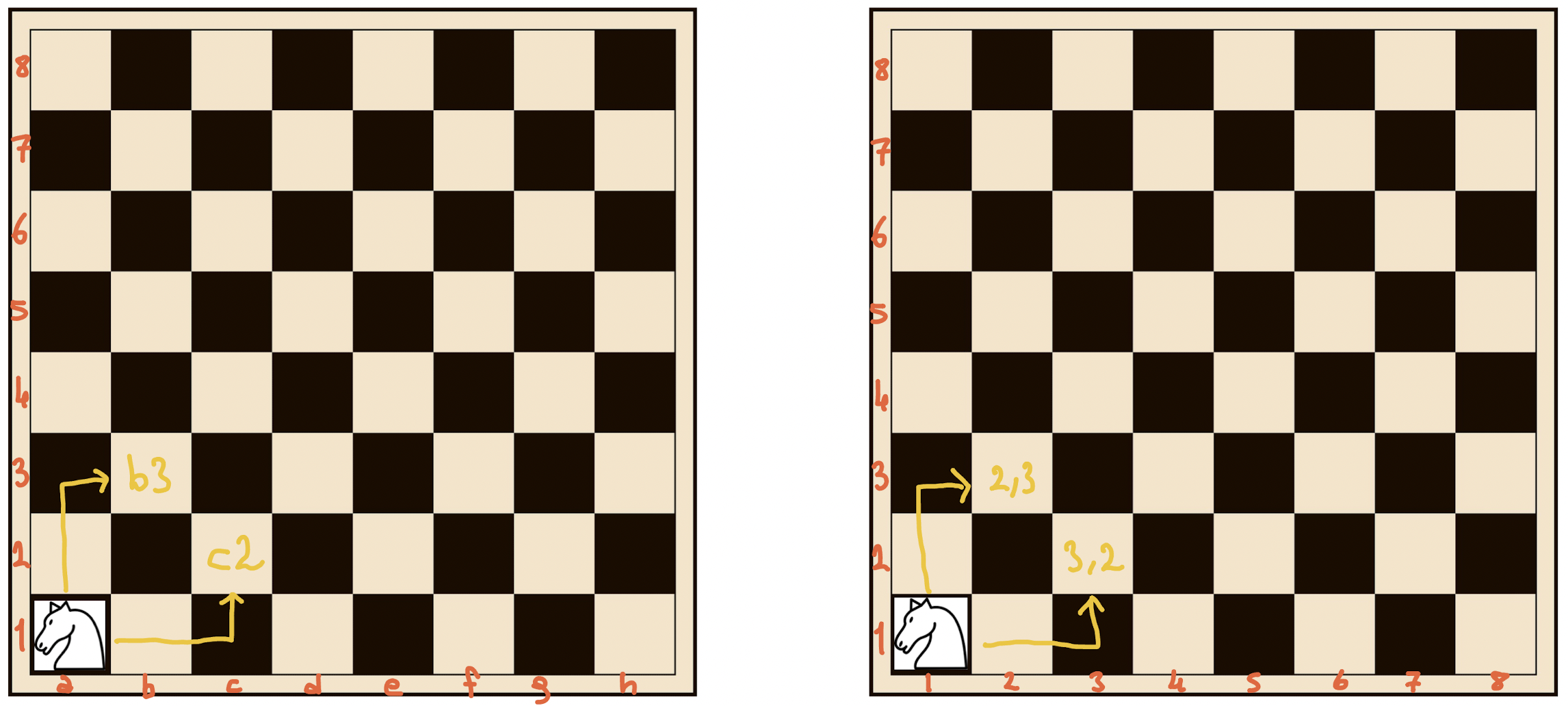## Posts

Showing posts from August, 2022

### Chess Knight Possible Moves Algorithm with DrawingAt first, I will define and explain the algorithm and its solution with a drawing. At the end of the post, I will show you how I solve the algorithm in Java. Problem Our algorithm will progress on a classic 8x8 chessboard that is empty. We will give the algorithm the abscissa and ordinate of the position where we put the knight, and the algorithm will try to find out how many different points the knight can move in this position. Solution The chess coordinates are like the picture on the left; b3, c2, and so on. But when solving the knight jump algorithm, we will think like the picture on the right. The abscissa and ordinate will be numeric values such as (1, 1) or (2, 3). Why do we think this way? Let's explain with a Coordinate System. Let's place this Coordinate System on the knight, like below. But for easier understanding, let's first place it in the area where the knight can move the most move on the chessboard. Now, you can see the knight can 8 different moves. Also,